Constructing Quadrilateral - 4 sides and diagonal given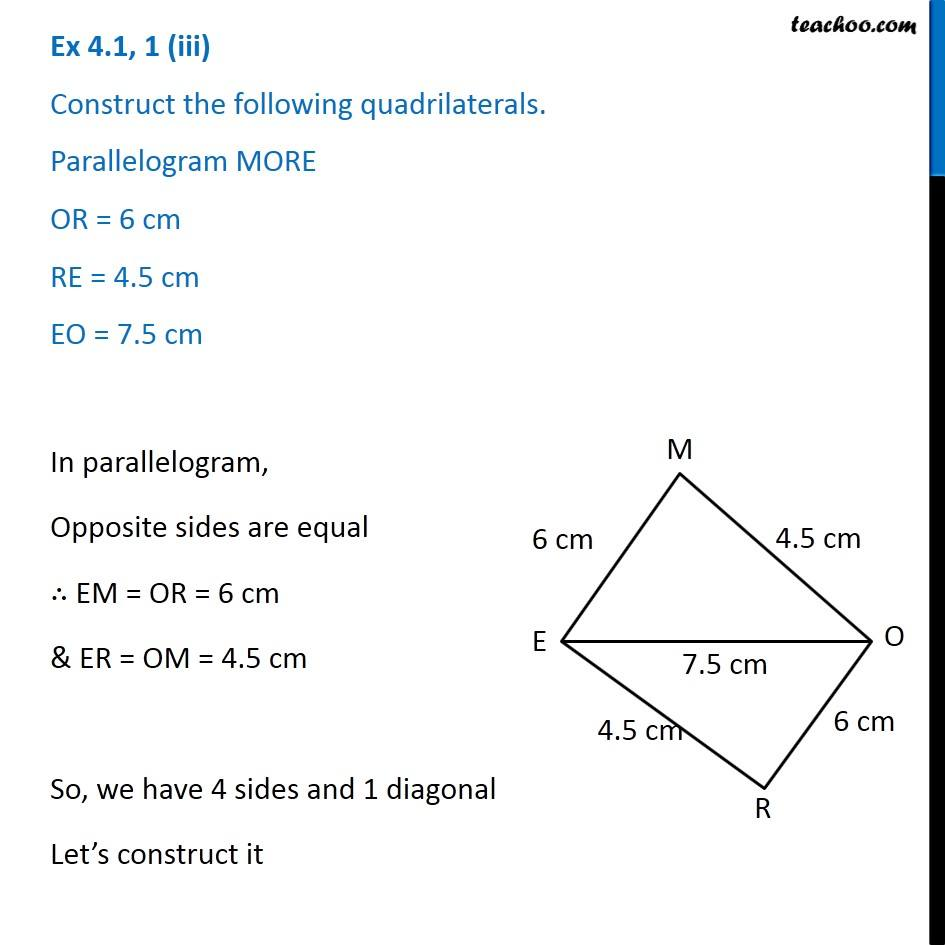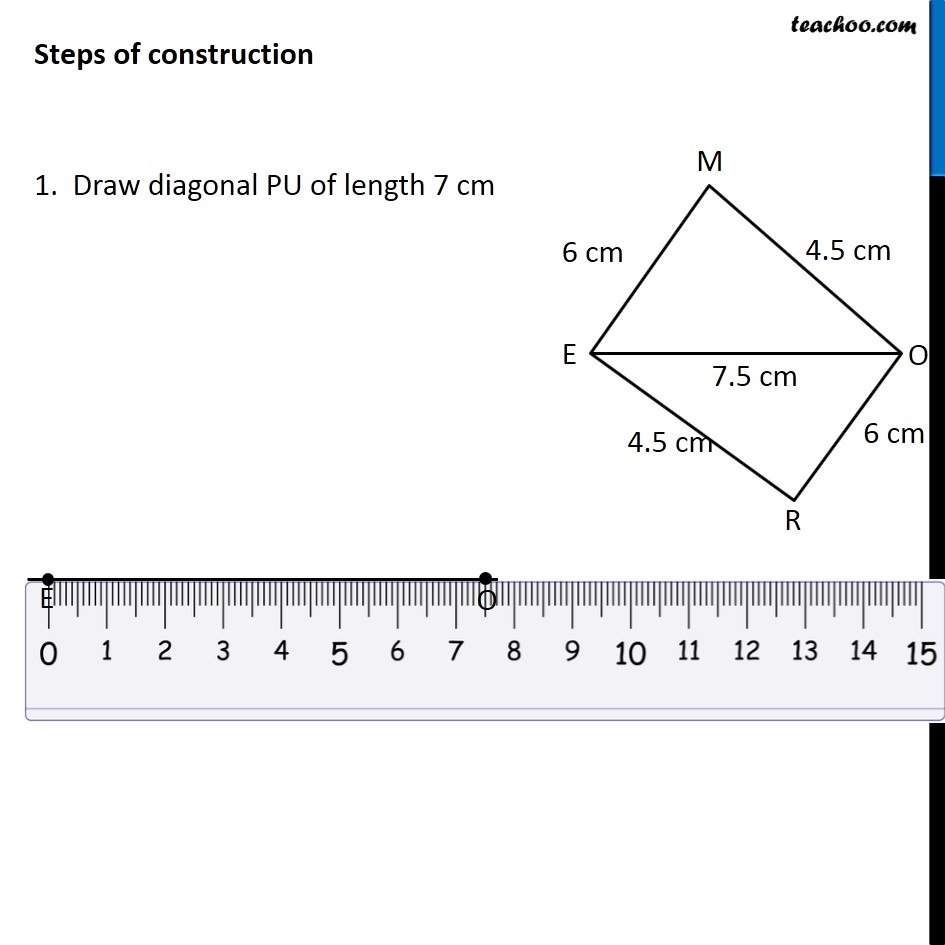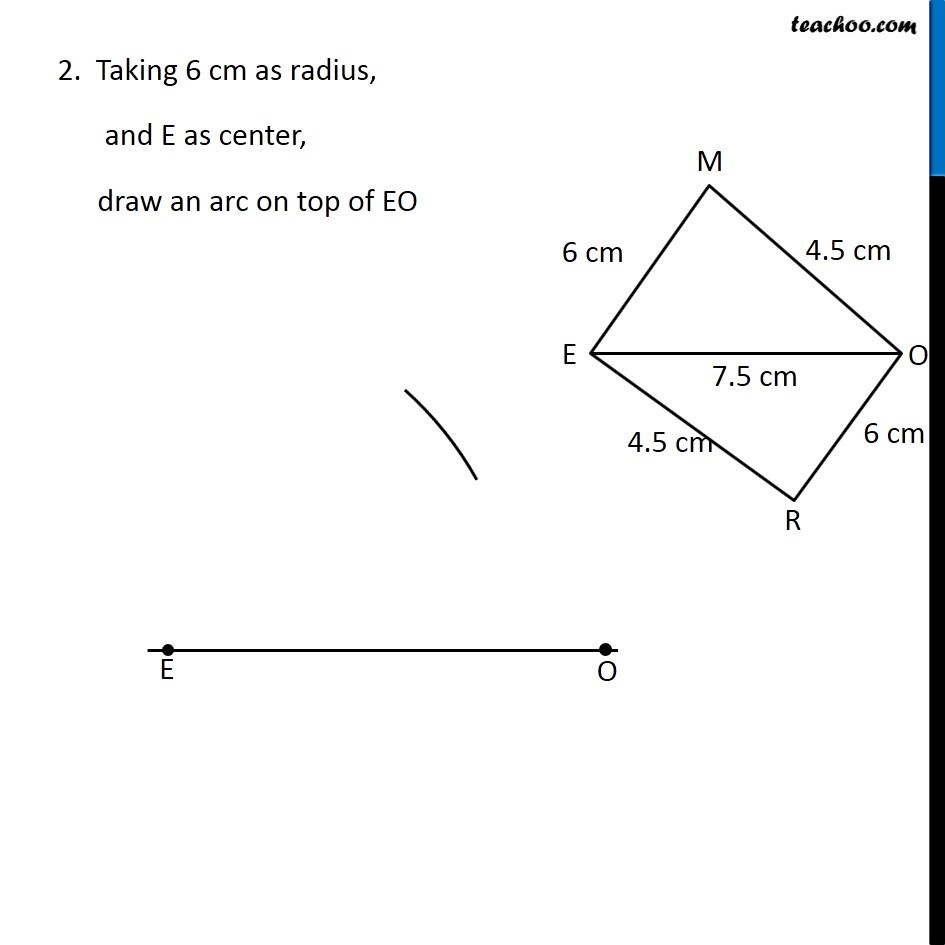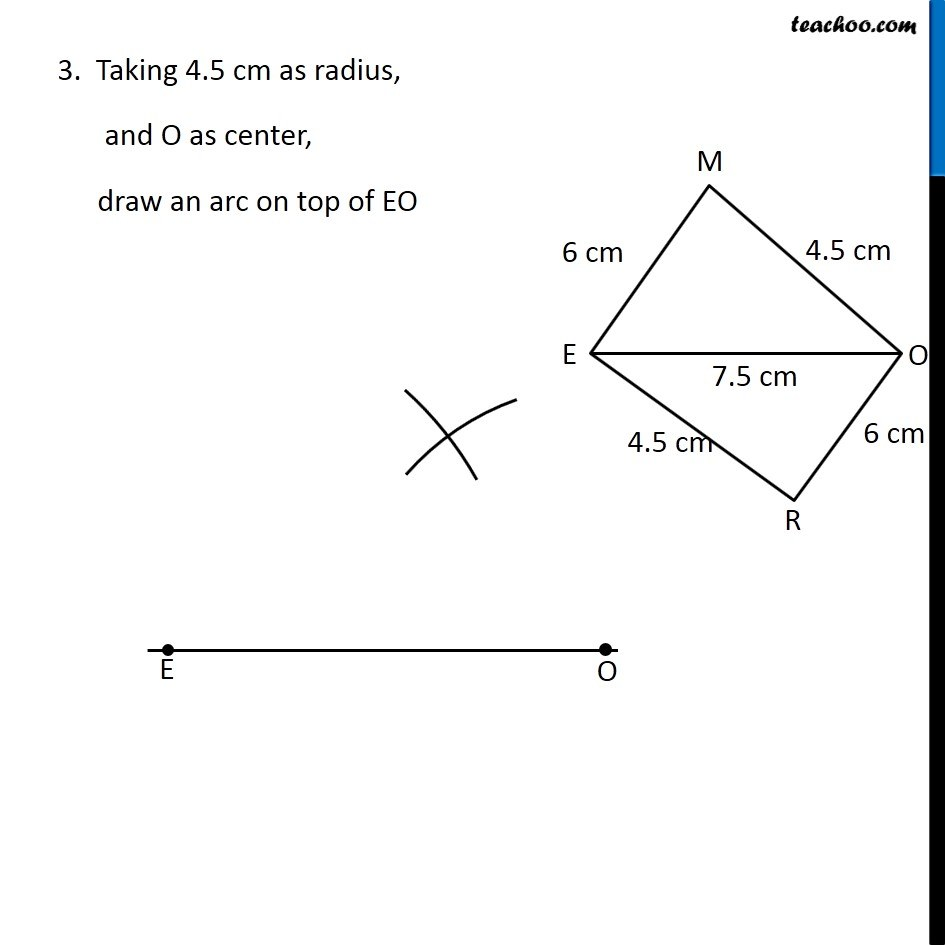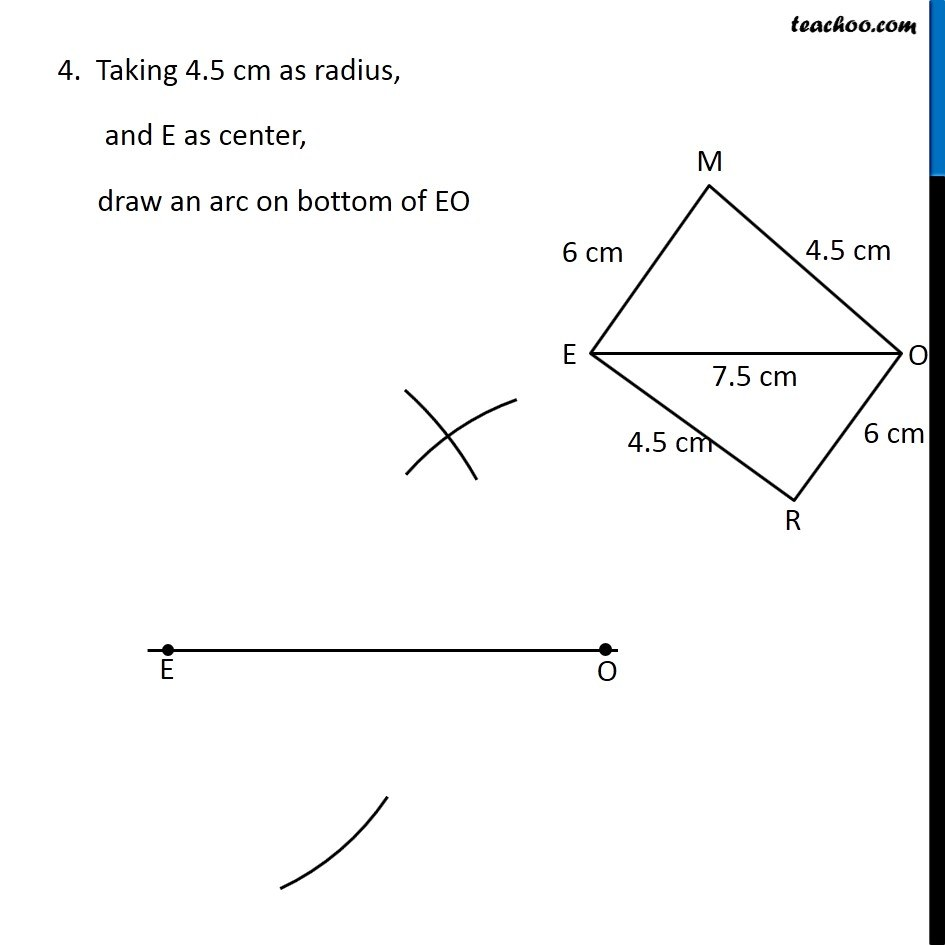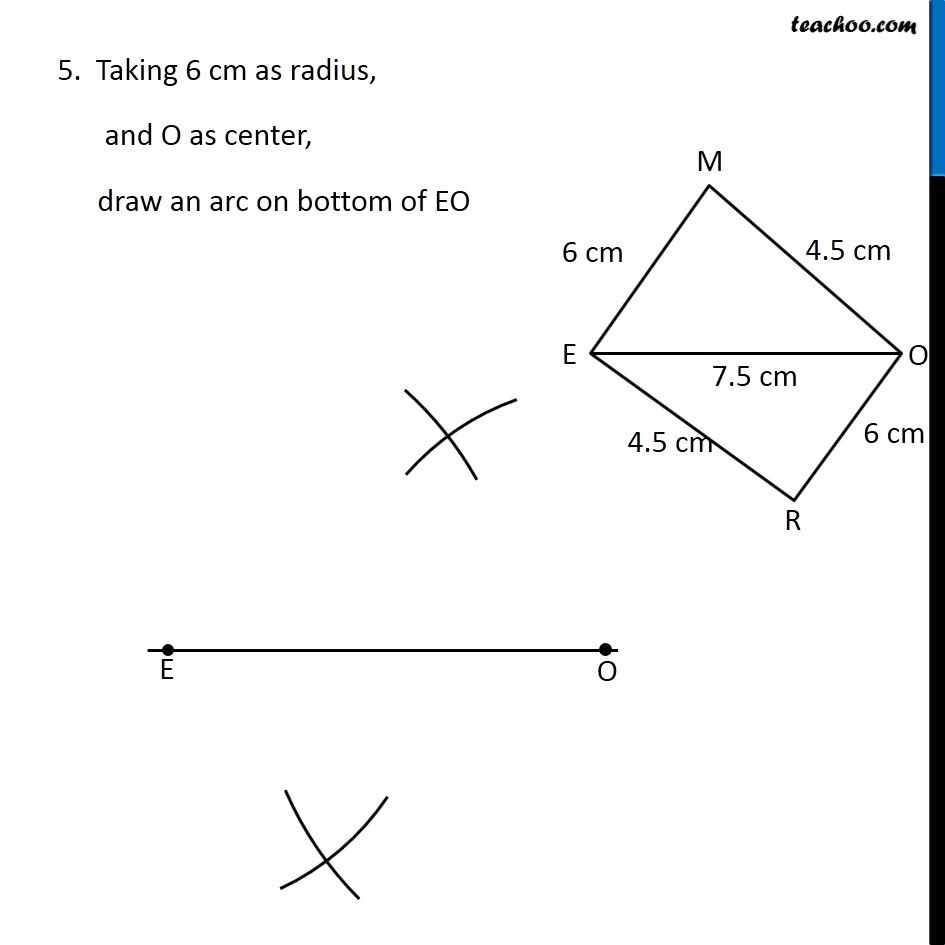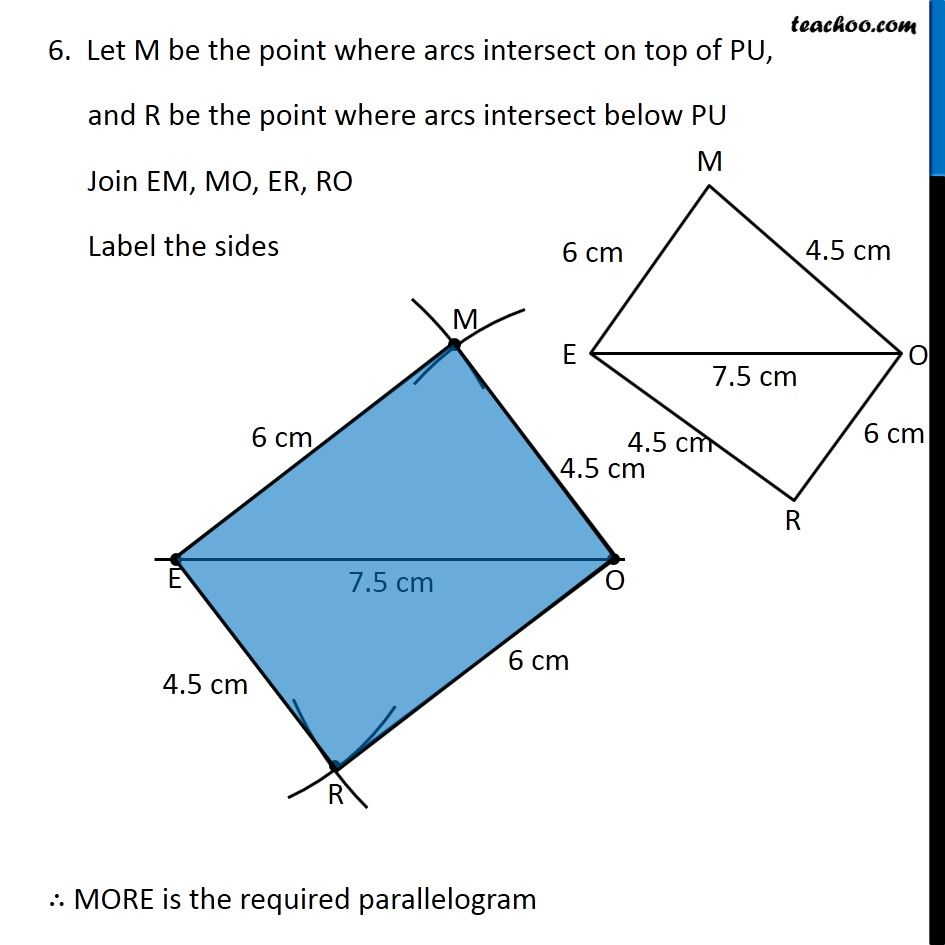Learn in your speed, with individual attention - Teachoo Maths 1-on-1 Class

### Transcript

Question 1 (iii) Construct the following quadrilaterals. Parallelogram MORE OR = 6 cm RE = 4.5 cm EO = 7.5 cm In parallelogram, Opposite sides are equal ∴ EM = OR = 6 cm & ER = OM = 4.5 cm So, we have 4 sides and 1 diagonal Let’s construct it Steps of construction 1. Draw diagonal PU of length 7 cm 2. Taking 6 cm as radius, and E as center, draw an arc on top of EO 3. Taking 4.5 cm as radius, and O as center, draw an arc on top of EO 4. Taking 4.5 cm as radius, and E as center, draw an arc on bottom of EO 5. Taking 6 cm as radius, and O as center, draw an arc on bottom of EO 6. Let M be the point where arcs intersect on top of PU, and R be the point where arcs intersect below PU Join EM, MO, ER, RO Label the sides ∴ MORE is the required parallelogram